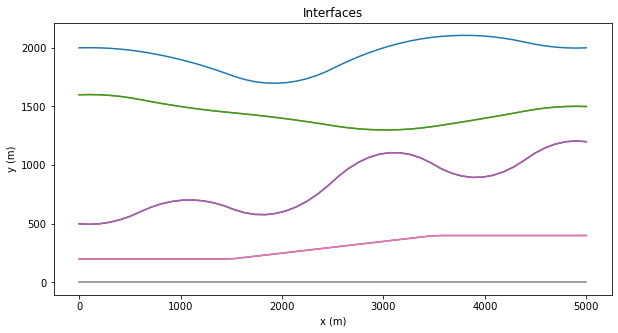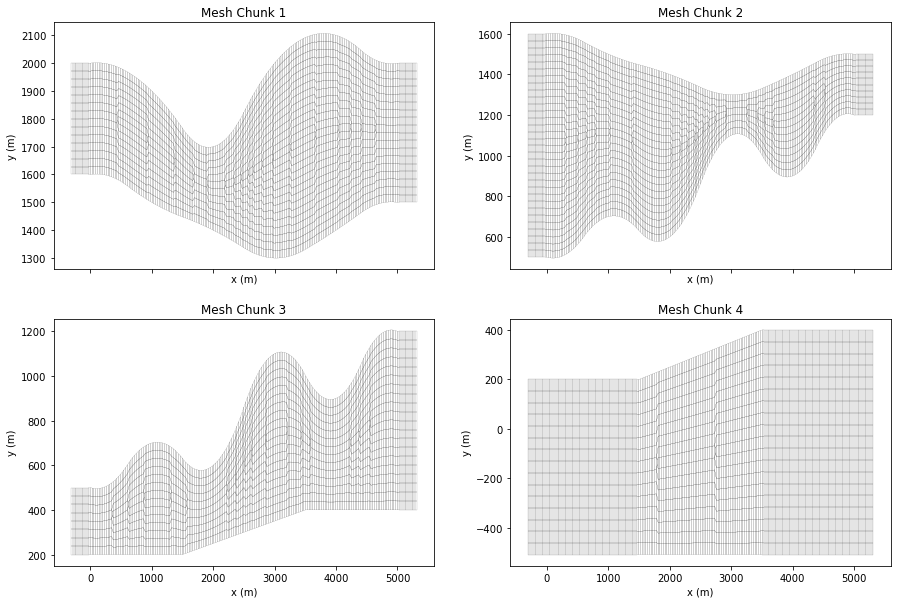Version:

This documentation is not for the latest stable Salvus version.This tutorial is presented as Python code running inside a Jupyter Notebook, the recommended way to use Salvus. To run it yourself you can copy/type each individual cell or directly download the full notebook, including all required files.# Modelling Layered Topography

Copy
# Magic
%matplotlib inline
%config Completer.use_jedi = False

## Introduction

A common use case for spectral-element simulations is for the simulation of wavefields in the presence of surface, subsurface, or seabed topography. In this tutorial we'll

• Define a set set of interfaces with topography by using interpolating splines.
• Generate a mesh which respects the defined topography.
• Set up and run an acoustic simulation through the resulting mesh.

Let's get started by importing the relevant Python packages.

import os
import matplotlib.pyplot as plt
import numpy as np

import salvus.flow.simple_config as config
import salvus.toolbox.toolbox as st
from salvus.flow import api
from salvus.flow import simple_config as config
from salvus.mesh.optimize_dt import optimize_dt
from salvus.mesh.simple_mesh import rho_from_gardeners, vs_from_poisson

SALVUS_FLOW_SITE_NAME = os.environ.get("SITE_NAME", "local")

## Setting up the model

### Defining the interpolating splines

The first thing we'll do is set up the splines which will define the interfaces in our model. Before we get started though, let's set the x extent of our model, which will be $5$ km.

x_min = 0.0
x_max = 5000.0

To help topography, we'll make use of the get_interpolating_splines function from SalvusToolbox. This function requires a set of interpolation points which can be used to anchor the splines, and these points in turn are defined by a set of locations in $x$ and $y$. In the cell below we define a series of $5$ discontinuities by specifying their interpolation points in each coordiante. We multiply by $1000$ here simply for brevity.

layers_x = [
np.array([0.0, 0.2, 1.0, 2.0, 3.0, 4.0, 4.8, 5.0]) * 1000,
np.array([0.0, 0.2, 1.0, 2.0, 3.0, 4.0, 4.8, 5.0]) * 1000,
np.array([0.0, 0.2, 1.0, 2.0, 3.0, 4.0, 4.8, 5.0]) * 1000,
np.array([0.0, 1.5, 3.5, 5.0]) * 1000,
np.array([0.0, 2.5, 5.0]) * 1000,
]

layers_y = [
np.array([2.0, 2.0, 1.9, 1.7, 2.0, 2.1, 2.0, 2.0]) * 1000,
np.array([1.6, 1.6, 1.5, 1.4, 1.3, 1.4, 1.5, 1.5]) * 1000,
np.array([0.5, 0.5, 0.7, 0.6, 1.1, 0.9, 1.2, 1.2]) * 1000,
np.array([0.2, 0.2, 0.4, 0.4]) * 1000,
np.array([0.0, 0.0, 0.0]) * 1000,
]

We'll also define the p-wave velocities for each layer, and use some helper functions from Salvus Mesh to convert these values to realistic density and s-wave velocities.

# Define p-velocities.
vp = np.array([2000.0, 2500.0, 2800.0, 3200.0])

# Compute vs and rho.
vs = vs_from_poisson(vp)
rho = rho_from_gardeners(vp)

The get_interpolating_splines function also accepts an array of interpolations methods. Internally the function uses interp1d from Scipy, so please check out the documentation here for a complete list of interpolation styles.

interpolation_styles = [
"linear",
"linear",
]

Now we're ready to go ahead and generate our interpolating splines. Given the variables we defined above, we can call the function below to get the horizons of our model.

splines = st.get_interpolating_splines(
layers_x, layers_y, kind=interpolation_styles
)

Below we simply just plot the splines to get an idea of what our model will look like in the end. Indeed the extents and horizons look as we expect, and we can move on to generating the mesh itself. Note that none of the horizons here cross each other, and if this were the case than the subsequent mesh generation would fail with an error. We'll re-visit how to deal with pinched-out layers and crossing horizons in a future tutorial.

# Plot the interfaces.
f = plt.figure(figsize=(10, 5))
x_plot = np.linspace(x_min, x_max)
for top, bot in splines:
plt.plot(x_plot, top(x_plot))
plt.plot(x_plot, bot(x_plot))

plt.xlabel("x (m)")
plt.ylabel("y (m)")
plt.title("Interfaces")
Text(0.5, 1.0, 'Interfaces')### Generating the mesh

With our interfaces defined, we can now go ahead and generate the mesh itself. For this case a wrapper is included in the SalvusToolbox. This wrapper takes a series of parameters and attempts to build an optimal mesh with topography, and will also mark each individual region with a flag so it can be identified later. A more detailed description of the arguments one can pass is given below:

• x_min, x_max: The minimum and maximum x-values defining the model's horizontal extent. These are the dimensions to be considered before the attachment of absorbing boundaries. If absorbing boundaries are attached the resulting extent of the mesh will grow beyond x_min and x_max, but the solution will not be physical in the extruded regions.
• splines: The list of interpolation objects which we have generated above. These could of course be generated independently as well as long as their form is the same.
• absorbing_boundaries: A tuple consisting of the side sets which will be made absorbing, along with the number of elements to be extruded in each dimension for the absorbing layer. As previously stated, we recommend an absorbing layer of 3.5+ wavelengths if minimal reflections are desired.
• slowest_velocities: A list of the slowest velocities in each layer, ordered the same as the interfaces passed through splines (i.e. top to bottom). These values will be used, along with maximum_frequency and elements_per_wavelength to determine the element sizes in each region.
• use_refinements: Create a totally unstrucuted mesh by allowing for vertical refinements. Whether or not this will result in a performance increase is dependent on the model, we recommend try with and without refinements to see what works best for you.
• maximum_frequency: The maximum frequency to resolve in the mesh, which will be used in conjunction with elements_per_wavelength.
# Maximum frequency to resolve with elements_per_wavelength.
max_frequency = 20.0

# Generate the mesh
mesh, bnd = st.generate_mesh_from_splines_2d(
x_min=0,
x_max=x_max,
splines=splines,
elements_per_wavelength=2,
maximum_frequency=max_frequency,
use_refinements=True,
slowest_velocities=vs,
absorbing_boundaries=(["x0", "x1", "y0"], 10.0),
)

This function has returned a tuple of two elements. The first entry contains a list of mesh chunks, each of which correspond to a separate region in between two of the defined horizons. The second value returns the minimum size in meters of the extruded boundaries. This value will be used later on when running the actual simulation. We can plot the mesh chunks individually using the build in plot functionality of the mesher. Keep in mind that this may take some time for bigger meshes, so if you are running at higher frequencies you may want to skip of comment out the following cell.

f, axs = plt.subplots(2, 2, figsize=(15, 10), sharex=True)
for _i, (ax, sub_mesh) in enumerate(zip(axs.flatten(), mesh)):

plt.sca(ax)
ax.set_xlabel("x (m)")
ax.set_ylabel("y (m)")
ax.set_title(f"Mesh Chunk {_i + 1}")
sub_mesh.plot(show=False, figure=f, linewidths=0.1)Our four individual mesh chunks are now properly properly sized and deformed, and are ready to be glued together. Each individual chunk has an elemental variable called region attached to it, and we'll use this variable later on to track which region is which when we're attaching parameters. To sum the mesh regions into one continuous mesh all we need to do is simply call the sum operation on the array of sub-meshes as below...

mesh = np.sum(mesh)

...and now the meshing stage is complete. The cell below will visualize the complete mesh. Note that we have the usual side sets (x0, x1, y0, y1) on the edges of our mesh, but we also have additional side sets which mark the internal discontinuities. These internal side sets can be handy when placing sources and receivers, as we'll explore in a future tutorial.

mesh  # Visualize the mesh.